# Electric field

ElectromagnetismElectricity ·Magnetism
Electrostatics
Coulomb’s law · Electric field · Electric flux · Gauss’ law · Electric potential · Electrostatic induction · Electric dipole moment ·

In physics, the space surrounding an electric charge or in the presence of a time-varying magnetic field has a property called an electric field (that can also be equated to electric flux density). This electric field exerts a force on other electrically charged objects. The concept of electric field was introduced by Michael Faraday.

The electric field is a vector field with SI units of newtons per coulomb (N C−1) or, equivalently, volts per meter (V m−1). The strength of the field at a given point is defined as the force that would be exerted on a positive test charge of +1 coulomb placed at that point; the direction of the field is given by the direction of that force. Electric fields contain electrical energy with energy density proportional to the square of the field intensity. The electric field is to charge as gravitational acceleration is to mass and force density is to volume.

A moving charge has not just an electric field but also a magnetic field, and in general the electric and magnetic fields are not completely separate phenomena; what one observer perceives as an electric field, another observer in a different frame of reference perceives as a mixture of electric and magnetic fields. For this reason, one speaks of "electromagnetism" or "electromagnetic fields." In quantum mechanics, disturbances in the electromagnetic fields are called photons, and the energy of photons is quantized.

## Definition

A stationary charged particle in an electric field experiences a force proportional to its charge given by the equation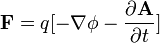$\mathbf{F} = q[- \nabla \phi - \frac { \partial \mathbf{A} } { \partial t }]$

where the magnetic flux density is given by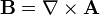$\mathbf{B} = \nabla \times \mathbf{A}$

and where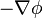$- \nabla \phi$ is the Coulomb force. (See the section below).

Electric charge is a characteristic of some subatomic particles, and is quantized when expressed as a multiple of the so-called elementary charge e. Electrons by convention have a charge of -1, while protons have the opposite charge of +1. Quarks have a fractional charge of −1/3 or +2/3. The antiparticle equivalents of these have the opposite charge. There are other charged particles.

In general, same-sign charged particles repel one another, while different-sign charged particles attract. This is expressed quantitatively in Coulomb's law, which states the magnitude of the repelling force is proportional to the product of the two charges, and weakens proportionately to the square of the distance.

The electric charge of a macroscopic object is the sum of the electric charges of its constituent particles. Often, the net electric charge is zero, since naturally the number of electrons in every atom is equal to the number of the protons, so their charges cancel out. Situations in which the net charge is non-zero are often referred to as static electricity. Furthermore, even when the net charge is zero, it can be distributed non-uniformly (e.g., due to an external electric field), and then the material is said to be polarized, and the charge related to the polarization is known as bound charge (while the excess charge brought from outside is called free charge). An ordered motion of charged particles in a particular direction (in metals, these are the electrons) is known as electric current. The discrete nature of electric charge was proposed by Michael Faraday in his electrolysis experiments, then directly demonstrated by Robert Millikan in his oil-drop experiment.

The SI unit for quantity of electricity or electric charge is the coulomb, which represents approximately 1.60 × 1019 elementary charges (the charge on a single electron or proton). The coulomb is defined as the quantity of charge that has passed through the cross-section of an electrical conductor carrying one ampere within one second. The symbol Q is often used to denote a quantity of electricity or charge. The quantity of electric charge can be directly measured with an electrometer, or indirectly measured with a ballistic galvanometer.

Formally, a measure of charge should be a multiple of the elementary charge e (charge is quantized), but since it is an average, macroscopic quantity, many orders of magnitude larger than a single elementary charge, it can effectively take on any real value. Furthermore, in some contexts it is meaningful to speak of fractions of a charge; e.g. in the charging of a capacitor.

If the charged particle can be considered a point charge, the electric field is defined as the force it experiences per unit charge: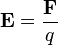$\mathbf{E} = \frac{\mathbf{F}}{q}$

where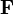$\mathbf{F}$ is the electric force experienced by the particle
q is its charge$\mathbf{E}$ is the electric field wherein the particle is located

Taken literally, this equation only defines the electric field at the places where there are stationary charges present to experience it. Furthermore, the force exerted by another charge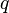$q$ will alter the source distribution, which means the electric field in the presence of$q$ differs from itself in the absence of$q$. However, the electric field of a given source distribution remains defined in the absence of any charges with which to interact. This is achieved by measuring the force exerted on successively smaller test charges placed in the vicinity of the source distribution. By this process, the electric field created by a given source distribution is defined as the limit as the test charge approaches zero of the force per unit charge exerted thereupon.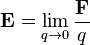$\mathbf{E}=\lim_{q \to 0}\frac{\mathbf{F}}{q}$

This allows the electric field to be dependent on the source distribution alone.

As is clear from the definition, the direction of the electric field is the same as the direction of the force it would exert on a positively-charged particle, and opposite the direction of the force on a negatively charged particle. Since like charges repel and opposites attract (as quantified below), the electric field tends to point away from positive charges and towards negative charges.

## Coulomb's law

The electric field surrounding a point charge is given by Coulomb's law: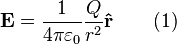$\mathbf{E} =\frac{1}{4 \pi \varepsilon_0}\frac{Q}{r^2}\mathbf{\hat{r}} \qquad \mbox{(1)}$

where

Q is the charge of the particle creating the electric field,
r is the distance from the particle with charge Q to the E-field evaluation point,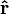$\mathbf{\hat{r}}$ is the Unit vector pointing from the particle with charge Q to the E-field evaluation point,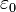$\varepsilon_0$ is the vacuum permittivity.

Coulomb's law is actually a special case of Gauss's Law, a more fundamental description of the relationship between the distribution of electric charge in space and the resulting electric field. Gauss's law is one of Maxwell's equations, a set of four laws governing electromagnetics.

## Time-varying fields

Charges do not only produce electric fields. As they move, they generate magnetic fields, and if the magnetic field changes, it generates electric fields. A changing magnetic field gives rise to an electric field,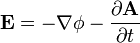$\mathbf{E} = - \nabla \phi - \frac { \partial \mathbf{A} } { \partial t }$

which yields Faraday's law of induction,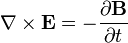$\nabla \times \mathbf{E} = -\frac{\partial \mathbf{B}} {\partial t}$

where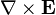$\nabla \times \mathbf{E}$ indicates the curl of the electric field,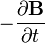$-\frac{\partial \mathbf{B}} {\partial t}$ represents the vector rate of decrease of magnetic field with time.

This means that a magnetic field changing in time produces a curled electric field, possibly also changing in time. The situation in which electric or magnetic fields change in time is no longer electrostatics, but rather electrodynamics or electromagnetics.

## Properties (in electrostatics)

According to equation (1) above, electric field is dependent on position. The electric field due to any single charge falls off as the square of the distance from that charge.

Electric fields follow the superposition principle. If more than one charge is present, the total electric field at any point is equal to the vector sum of the respective electric fields that each object would create in the absence of the others.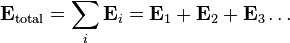$\mathbf{E}_{\rm total} = \sum_i \mathbf{E}_i = \mathbf{E}_1 + \mathbf{E}_2 + \mathbf{E}_3 \ldots \,\!$

If this principle is extended to an infinite number of infinitesimally small elements of charge, the following formula results: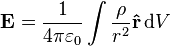$\mathbf{E} = \frac{1}{4\pi\varepsilon_0} \int\frac{\rho}{r^2} \mathbf{\hat{r}}\,\mathrm{d}V$

where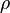$\rho$ is the charge density, or the amount of charge per unit volume.

The electric field at a point is equal to the negative gradient of the electric potential there. In symbols,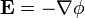$\mathbf{E} = -\nabla \phi$

where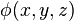$\phi(x, y, z)$ is the scalar field representing the electric potential at a given point.

If several spatially distributed charges generate such an electric potential, e.g. in a solid, an electric field gradient may also be defined.

Considering the permittivity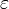$\varepsilon$ of a material, which may differ from the permittivity of free space$\varepsilon_{0}$, the electric displacement field is: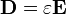$\mathbf{D} = \varepsilon \mathbf{E}$

## Energy in the electric field

The electric field stores energy. The energy density of the electric field is given by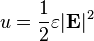$u = \frac{1}{2} \varepsilon |\mathbf{E}|^2$

where$\varepsilon$ is the permittivity of the medium in which the field exists$\mathbf{E}$ is the electric field vector.

The total energy stored in the electric field in a given volume V is therefore$\int_{V} \frac{1}{2} \varepsilon |\mathbf{E}|^2 \, \mathrm{d}V$

where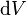$\mathrm{d}V$ is the differential volume element.

## Parallels between electrostatics and gravity

Coulomb's law, which describes the interaction of electric charges: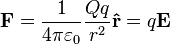$\mathbf{F} = \frac{1}{4 \pi \varepsilon_0}\frac{Qq}{r^2}\mathbf{\hat{r}} = q\mathbf{E}$

is similar to the Newtonian gravitation law: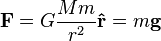$\mathbf{F} = G\frac{Mm}{r^2}\mathbf{\hat{r}} = m\mathbf{g}$

This suggests similarities between the electric field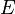$E$ and the gravitational field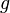$g$, so sometimes mass is called "gravitational charge."

Similarities between electrostatic and gravitational forces:

1. Both act in a vacuum.
2. Both are central and conservative.
3. Both obey an inverse-square law (both are inversely proportional to square of r).
4. Both propagate with finite speed c.

Differences between electrostatic and gravitational forces:

1. Electrostatic forces are much greater than gravitational forces (by about 1036 times).
2. Gravitational forces are attractive for like charges, whereas electrostatic forces are repulsive for like charges.
3. There are no negative gravitational charges (no negative mass) while there are both positive and negative electric charges. This difference combined with previous implies that gravitational forces are always attractive, while electrostatic forces may be either attractive or repulsive.
4. Electric charge is invariant while relativistic mass is not.